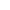Physics | ICSE Board | Grade 12 | 2016
Q. In a nuclear reactor, what is the function of a moderator?
View SolutionQ. A coil has a self-inductance of 0.05 henry. Find magnitude of the emf induced in it when the current flowing through it is changing at the rate 100As1.
View SolutionQ. Calculate angular momentum of an electron in the third Bohr orbit of hydrogen atom.
View SolutionQ. Name the principle on the basis of which optical fibres work.
View SolutionQ. What is the difference between polarised light and unpolarised light?
View SolutionQ. Two metals A and B have work functions 4eV and 6eV respectively. Which metal has lower threshold wavelength for photoelectric effect?
View SolutionQ. Total energy of an electron in the ground state of hydrogen atom is 13.6eV. Its total energy, when hydrogen atom is in the first excited state, is:
1. +13.6eV
2. +3.4eV
3. 3.4eV
4. 54.4eV
View SolutionQ. In our Nature, where is the nuclear fusion reaction taking place continuously?
View SolutionQ. i. State Gauss' law.
ii. In an electric dipole, at which point is the electric potential zero?
View SolutionQ. For a metallic conductor, what is the relation between current density (j), conductivity (σ) and electric field Intensity E?
View SolutionQ. Obtain an expression for equivalent capacitance when three capacitors C1, C2 and C3 are connected in series.
View SolutionQ. i. Define Curie temperature.
ii. If magnetic susceptibility of a certain magnetic material is 0.0001, find its relative permeability.
View SolutionQ. A charged oil drop weighing 1.6×1015N is found to remain suspended in a uniform electric field of intensity 2×103NC1. Find the charge on the drop.
View SolutionQ. State how a moving coil galvanometer can be converted into an ammeter.
View SolutionQ. Calculate dispersive power of a transparent material with the given:
nv=1.56, nr=1.54, ny=1.55
View SolutionQ. Write the expression for the Lorentz force F in vector form.
View SolutionQ. A carbon resistor has coloured bands as shown in Figure given below. The resistance of the resistor is:1. 26Ω±10%
2. 26Ω±5%
3. 260Ω±5%
4. 260Ω±10%
View SolutionQ. If current through the conductor Y is reversed in direction, will neutral point lie between X and Y, to the left of X or to the right of Y?View SolutionQ. In figure given above, find the value of resistance X for which points A and B are at the same potential :View SolutionQ. A solenoid L and a resistor R are connected in series to a battery, through a switch. When the switch is put on, current I flowing through it varies with time t as shown in which of the graphs given below:
1.2.3.4.View SolutionQ. In the circuit shown in Figure above, E1 and E2 are two cells having emfs 2V and 3V respectively, and negligible internal resistances. Applying Kirchhoff's laws of electrical networks, find the values of currents I1 and I2.View SolutionQ. Two point charges Q1=400μC and Q2=100μC are kept fixed, 60 cm apart in vacuum. Find intensity of the electric field at midpoint of the line joining Q1 and Q2.
View SolutionQ. Draw a labelled circuit diagram of a potentiometer to measure internal resistance of a cell. Write the working formula. (Derivation not required).
View SolutionQ. What is meant by short-sightedness?
View SolutionQ. In given Figure, a charge Q is fixed. Another charge q is moved along a circular arc MN of radius r around it, from the point M to the point N such that the length of the arc MN=l. The work done in this process is :1. Zero
2. 14πϵ0.Qqr2l
3. Qq2ϵ0r2l
4. Qq2πϵ0r2
View SolutionQ. Two infinitely long current carrying conductors X and Y are kept parallel to each other, 24 cm apart in vacuum. They carry currents of 5A and 7A respectively, in the same direction, as shown in Figure above. Find the position of a neutral point, i.e. a point where resultant magnetic flux density is zero.
(Ignore earth's magnetic field).View SolutionQ. A metallic wire has a resistance of 3.0Ω at 0oC and 4.8Ω at 150oC. Find the temperature coefficient of resistance of its material.
View SolutionQ. Two thin lenses having optical powers of 10D and +6D are placed in contact with each other. The focal length of the combination is:
1. +0.25cm
2. 0.25cm
3. +0.25m
4. 0.25m
View SolutionQ. To which regions of the electromagnetic spectrum do the following wavelengths belong :
(a) 250nm
(b) 1500nm
View SolutionQ. What is the use of a Zener diode?
View Solution